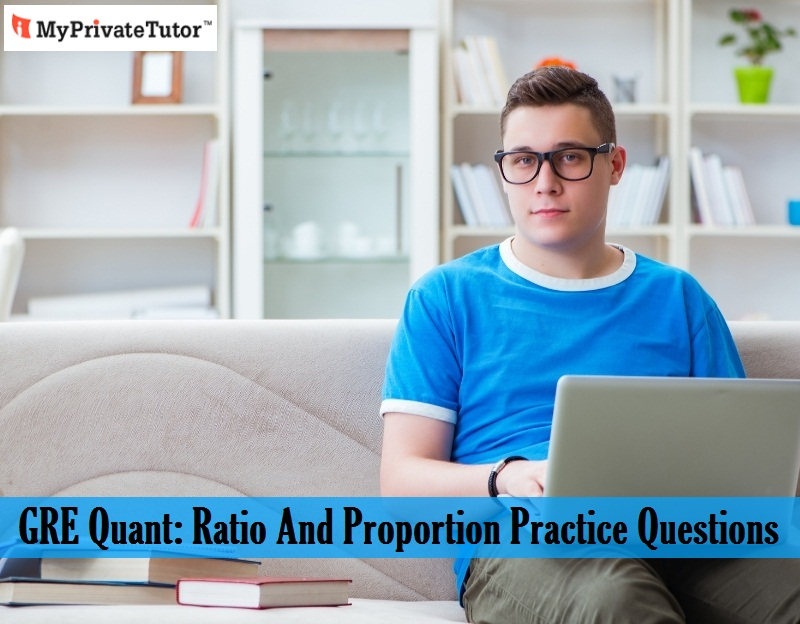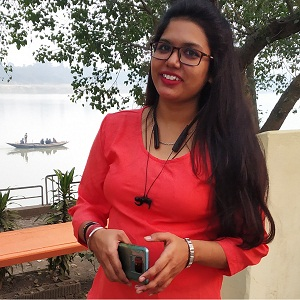# GRE Quant: Ratio And Proportion Practice Questions

Jun 04, 2021GRE is one of the most important exams to take for MBA and other higher studies. GRE comes with three sections: Quantitative reasoning, verbal reasoning, and analytical writing. GRE quant and verbal sections come with MCQs. Analytical writing section, on the other hand, holds descriptive question. GRE quant is all about math and GRE verbal is all about English skills.

GRE quant includes different types of math questions and it includes a diverse array of topics from Arithmetic, algebra, geometry, and data analysis. Candidates mostly focus on difficult problems for this section. However, arithmetic portion of GRE quant consists of a few answerable questions and these don’t take much time to solve. GRE quant questions on ratio and proportion are among the easiest questions of GRE. A little practice with these questions will help the candidates to secure a point here.
Here are some questions and the solutions:

1. If the ratio of men to women at a party is 4:7, which of the following could be the number of people at the party?
A. 50
B. 64
C. 66
D. 70
E. 78
It may seem that there is not enough information to answer this, but to answer a problem like this, you just need to add the coefficient x to each quantity and add: 4x+7x=11x.
Here is the solution:
The sum of the quantities is 11 and here, it represents a fraction of the entire number of party-goers, therefore, the answer has to be a multiple of 11.
Among the answer options, the only multiple of 11 in our choices is 66 (option C).
Or you can can test it in the following way:
If 11x=66, then x = 6.
4x= 4*6= 24 - the number of men
7x=7*6=42 - the number of women

2. Find the combined ratio of (5 : 6), (7 : 9), (10 : 11).
A. 56/157
B. 65/99
C. 21/31
D. 1/5
If we compound or compile two or more ratio, then, a : b and c : d, it will become ac:bd.
Therefore, the calculation as follows
(5 : 6), (7 : 9), (10 : 11) = 5/6 * 7/9 * 10/11 = 350/594
= 65/99 (option B)

3. A mixture of sugar and water is in the ratio 3 : 2. A man adds 9 liters of water, and the mixture comes in the ratio of 3 : 5. Find the quantity of sugar in the new mixture.
A. 9
B. 15
C. 12
D. 10

Let’s assume water is 2x, and sugar is 3x.
Given, 3x/2x+9 = 3/5
15x = 6x + 27
9x = 27
x = 3
Therefore, quantity of sugar = 3 * 3 = 9 liters (option A)

4. Red, blue and yellow marbles in a bag have a ratio of 5 to 2 to 6. After removing the red marbles, there are 32 marbles left in the bag. How many red marbles were in the bag?
(A) 4
(B) 8
(C) 20
(D) 24
(E) 52

Here, we need to use the total number of marbles along with the ratio of blue plus yellow.
blue + yellow = marbles left
2x + 6x = 32
8x = 32, so x = 4.
Make sure you’re answering the question being asked. Here, the wrong answer options include x = 4, 2x = 8 blue marbles, 6x = 24 yellow marbles, and 32 + 20 = 52 marbles originally in the bag. Now, you can use x = 4 and the original 5 : 2 : 6 ratio to find the number of red marbles.
5x = 5 × 4 = 20
There were 20 red marbles in the bag (Option C).

5. Find the value of x. 35/84 = x/24
(A) 8
(B) 10
(C) 25
(D) 26
(E) 30

35 × 24 = 84x Cross multiply.
x = 35 × 24 /84 Divide both sides by 84.
x = 35 × 24/84 = 7 × 5 × 2 × 2 × 6/2 × 6 × 7 = 10 Factor and cancel to simplify the calculations.
Therefore, x = 10 (option B).

6. If 100 dollars can buy 0.07 grams of a rare radioactive material, how many grams can you buy with 106 dollars?
(A) 7
(B) 70
(C) 700
(D) 7000
(E) 70,000

0.07/100 = g/106
0.07 × 106 = 100g Cross multiply.
g = (0.07 × 102 × 104)/100 = (7 × 104)/102= 7 × 102 Divide both sides by 100 and simplify.
g = 700 (option C).

These questions are comparatively easier than the other algebraic, geometric, and data analysis problems. These questions take less time and candidates can surely secure a whole point for these questions. They can get a GRE tutor to practice this - Contact MyPrivateTutor today and get expert GRE tutors.

Article Posted in: Academics Maths Student Tips
Tags: GRE GRE test### Rachita

With a passion for writing, Rachita is in the field of content writing for more than 5 years. Here, she writes the blogs for MyPrivateTutor Singapore. Being a literature student, she has a strong addiction to reading as well. On a lazy day, she just needs a cozy setting and her favorite book or web series.